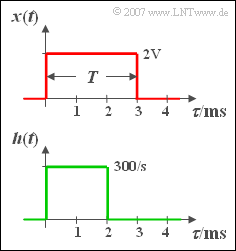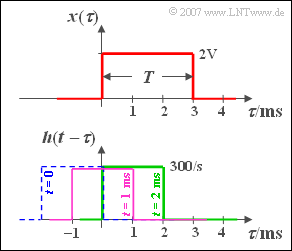# Exercise 3.8Z: Convolution of Two RectanglesThe convolution of two rectangles  $x(t)$  and  $h(t)$

At the input of a causal LTI system (i.e. linear and time-invariant)

• with a rectangular impulse response  ${h(t)}$  of duration  $2 \,\text{ms}$ ,
• a rectangular pulse  ${x(t)}$  of duration  $T = 3 \,\text{ms}$  and amplitude  $A = 2\,\text{ V}$  is applied.

The rectangular functions each start at the time  $t = 0$.

In this task you are to calculate the output signal  ${y(t)}$  with the help of the "Graphical Convolution".

As you can easily check, the output signal  ${y(t)}$

• differs from zero only in the range between  $0$  and  $5 \, \text{ms}$,  and
• is symmetrical at the time  $t = 2.5 \, \text{ms}$.

Hints:

### Questions

1

Calculate the signal values at the times   $t = 1 \,\text{ms}$  and  $t = 2 \,\text{ms}$.

 $y(t = 1 \,\text{ms})\ = \$  $\text{V}$ $y(t = 2 \,\text{ms})\ = \$  $\text{V}$

2

Determine the signal values for the times  $t = 3 \,\text{ms}$  and  $t = 4 \,\text{ms}$  by exploiting the symmetry properties.

 $y(t = 3 \,\text{ms})\ = \$  $\text{V}$ $y(t = 4 \,\text{ms})\ = \$  $\text{V}$

3

Which of the following statements are true?

 The output signal  ${y(t)}$  has a trapezoidal shape. The spectrum is   ${Y(f)} = Y_0 \cdot \text{si}^{2}(\pi f T)$. With  $T = 2 \,\text{ms}$,  a triangular shape would result.

### Solution

#### SolutionTo illustrate the convolution  $x(t) \star h(t)$;
the abscissas have been renamed:  $\tau$

(1)  In general, the following applies to the convolution integral:

$$y(t) = \int_{ - \infty }^{ + \infty } {x( \tau ) \cdot h( {t - \tau } )}\hspace{0.1cm} {\rm d}\tau.$$

The signal value at time  $t = 1 \,\text{ms}$  can be calculated as follows:

• Mirroring of the impulse response  ${h(\tau)}$,
• shifting by  $t = 1 \text{ ms}$  to the right (violet curve in the sketch),
• multiplication of the two functions and integration.

The product is also rectangular with the height  $2 \text{ V} \cdot 300 \; \text{1/s}$  and width  $1 \,\text{ms}$. This results for the area:

$$y( {t = 1\;{\rm{ms}}} ) \hspace{0.15 cm}\underline{= 0.6\;{\rm{V}}}{\rm{.}}$$

The green rectangle illustrates the calculation of the second signal value.  Now the resulting rectangle is twice as wide after the multiplication and we get:

$$y( {t = 2\;{\rm{ms}}} ) = 2\;{\rm{V}} \cdot {\rm{300}}\;{1}/{{\rm{s}}} \cdot 2\;{\rm{ms}}\hspace{0.15 cm}\underline{={\rm{1.2}}\;{\rm{V}}}{\rm{.}}$$

(2)  Because of the symmetry of  ${y(t)}$  with respect to the time  $t = 2.5\, \text {ms}$  holds:

$$y( {t = 3\;{\rm{ms}}} ) = y( {t = 2\;{\rm{ms}}} ) \hspace{0.15 cm}\underline{= {\rm{1}}{\rm{.2}}\;{\rm{V}}}{\rm{,}}$$
$$y( {t = 4\;{\rm{ms}}} ) = y( {t = 1\;{\rm{ms}}} )\hspace{0.15 cm}\underline{ = 0.6\;{\rm{V}}}{\rm{.}}$$

(3)  Proposed solutions 1 and 3 are correct:

• In subtasks  (1)  and  (2)  the signal values were calculated at discrete time points.
• All points are to be connected by straight line segments, since the integration over rectangular functions of increasing width results in a linear course.
• This means:  The output signal  ${y(t)}$  is trapezoidal.
• The associated spectrum is complex and reads:
$$Y(f) = 6 \cdot 10^{ - 3} \;{{\rm{V}}}/{{{\rm{Hz}}}} \cdot {\mathop{\rm si}\nolimits} ( {2\;{\rm{ms}}\cdot{\rm{\pi }}f} ) \cdot {\mathop{\rm si}\nolimits} ( {3\;{\rm{ms}}\cdot{\rm{\pi }}f}) \cdot {\rm{e}}^{ - {\rm{j \hspace{0.05cm}\cdot \hspace{0.05cm} 2 \hspace{0.05cm}\cdot \hspace{0.05cm}2.5\;{\rm{ms}}\hspace{0.05cm}\cdot \hspace{0.05cm} \pi }}f} .$$
• If the input pulse  ${x(t)}$  would have the duration  $T = 2\, \text {ms}$, the duration  ${y(t)}$  would show a triangular waveform between  ${t = 0}$  and  $t = 4 \text { ms}$.  The maximum  $1.2 \, \text {V}$  would then only result at the time  $t = 2 \, \text {ms}$.